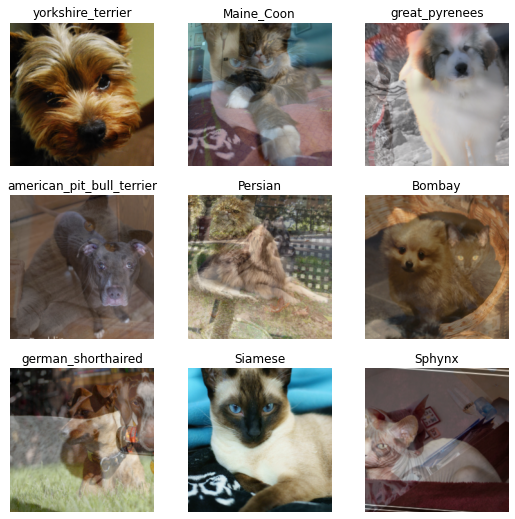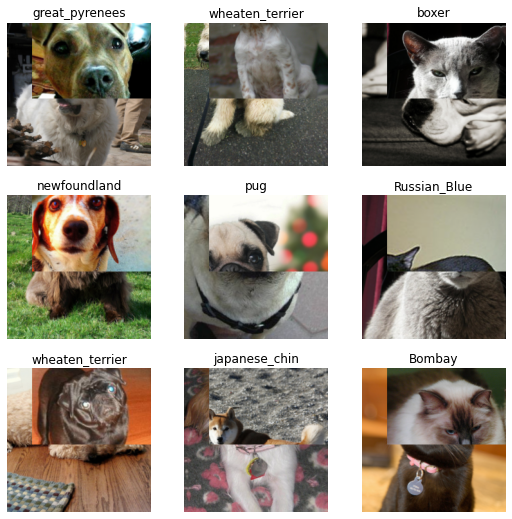# MixUp and Friends

Callbacks that can apply the MixUp (and variants) data augmentation to your training
from fastai.vision.all import *

source

### reduce_loss

 reduce_loss (loss:torch.Tensor, reduction:str='mean')

Reduce the loss based on reduction

Type Default Details
loss Tensor
reduction str mean PyTorch loss reduction
Returns Tensor

source

### MixHandler

 MixHandler (alpha:float=0.5)

A handler class for implementing MixUp style scheduling

Type Default Details
alpha float 0.5 Determine Beta distribution in range (0.,inf]

Most Mix variants will perform the data augmentation on the batch, so to implement your Mix you should adjust the before_batch event with however your training regiment requires. Also if a different loss function is needed, you should adjust the lf as well. alpha is passed to Beta to create a sampler.

source

### MixUp

 MixUp (alpha:float=0.4)

Implementation of https://arxiv.org/abs/1710.09412

Type Default Details
alpha float 0.4 Determine Beta distribution in range (0.,inf]

This is a modified implementation of mixup that will always blend at least 50% of the original image. The original paper calls for a Beta distribution which is passed the same value of alpha for each position in the loss function (alpha = beta = #). Unlike the original paper, this implementation of mixup selects the max of lambda which means that if the value that is sampled as lambda is less than 0.5 (i.e the original image would be <50% represented, 1-lambda is used instead.

The blending of two images is determined by alpha.

$$alpha=1.$$:

• All values between 0 and 1 have an equal chance of being sampled.
• Any amount of mixing between the two images is possible

$$alpha<1.$$:

• The values closer to 0 and 1 become more likely to be sampled than the values near 0.5.
• It is more likely that one of the images will be selected with a slight amount of the other image.

$$alpha>1.$$:

• The values closer to 0.5 become more likely than the numbers close to 0 or 1.
• It is more likely that the images will be blended evenly.

First we’ll look at a very minimalistic example to show how our data is being generated with the PETS dataset:

path = untar_data(URLs.PETS)
pat        = r'([^/]+)_\d+.*\$'
fnames     = get_image_files(path/'images')
item_tfms  = [Resize(256, method='crop')]
batch_tfms = [*aug_transforms(size=224), Normalize.from_stats(*imagenet_stats)]
dls = ImageDataLoaders.from_name_re(path, fnames, pat, bs=64, item_tfms=item_tfms,
batch_tfms=batch_tfms)

We can examine the results of our Callback by grabbing our data during fit at before_batch like so:

mixup = MixUp(1.)
with Learner(dls, nn.Linear(3,4), loss_func=CrossEntropyLossFlat(), cbs=mixup) as learn:
learn.epoch,learn.training = 0,True
learn.dl = dls.train
b = dls.one_batch()
learn._split(b)
learn('before_train')
learn('before_batch')

_,axs = plt.subplots(3,3, figsize=(9,9))
dls.show_batch(b=(mixup.x,mixup.y), ctxs=axs.flatten())
epoch train_loss valid_loss time
0 00:00We can see that every so often an image gets “mixed” with another.

How do we train? You can pass the Callback either to Learner directly or to cbs in your fit function:

learn = vision_learner(dls, resnet18, loss_func=CrossEntropyLossFlat(), metrics=[error_rate])
learn.fit_one_cycle(1, cbs=mixup)
epoch train_loss valid_loss error_rate time
0 2.041960 0.495492 0.162382 00:12

source

### CutMix

 CutMix (alpha:float=1.0)

Implementation of https://arxiv.org/abs/1905.04899

Type Default Details
alpha float 1.0 Determine Beta distribution in range (0.,inf]

Similar to MixUp, CutMix will cut a random box out of two images and swap them together. We can look at a few examples below:

cutmix = CutMix(1.)
with Learner(dls, nn.Linear(3,4), loss_func=CrossEntropyLossFlat(), cbs=cutmix) as learn:
learn.epoch,learn.training = 0,True
learn.dl = dls.train
b = dls.one_batch()
learn._split(b)
learn('before_train')
learn('before_batch')

_,axs = plt.subplots(3,3, figsize=(9,9))
dls.show_batch(b=(cutmix.x,cutmix.y), ctxs=axs.flatten())
epoch train_loss valid_loss time
0 00:00We train with it in the exact same way as well

learn = vision_learner(dls, resnet18, loss_func=CrossEntropyLossFlat(), metrics=[accuracy, error_rate])
learn.fit_one_cycle(1, cbs=cutmix)
epoch train_loss valid_loss accuracy error_rate time
0 3.440883 0.793059 0.769959 0.230041 00:12